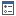﻿ NVector2DF Structure Properties
Nevron .NET Vision
Nevron.GraphicsCore Namespace / NVector2DF Structure

In This Topic
NVector2DF Structure Properties
In This Topic

For a list of all members of this type, see NVector2DF members.

Public Properties
NameDescriptionDetermines whether the vector is the unit vector (e.g. Length == 1)Gets the vector lenghtGets the vector lenght square (length * length)Gets the unit vector (with length == 1), which is perpendicular to this vectorGets a vector, which is perpendicular to this vector
Top